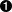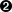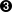## 2.7. Using lambda functions

Python supports an interesting syntax that lets you define one-line mini-functions on the fly. Borrowed from Lisp, these so-called lambda functions can be used anywhere a function is required.

### Example 2.21. Introducing lambda functions

```>>> def f(x):
...     return x*2
...
>>> f(3)
6
>>> g = lambda x: x*2>>> g(3)
6
>>> (lambda x: x*2)(3)6```This is a lambda function that accomplishes the same thing as the normal function above it. Note the abbreviated syntax here: there are no parentheses around the argument list, and the return keyword is missing (it is implied, since the entire function can only be one expression). Also, the function has no name, but it can be called through the variable it is assigned to.You can use a lambda function without even assigning it to a variable. Not the most useful thing in the world, but it just goes to show that a lambda is just an in-line function.

To generalize, a lambda function is a function that takes any number of arguments (including optional arguments) and returns the value of a single expression. lambda functions can not contain commands, and they can not contain more than one expression. Don’t try to squeeze too much into a lambda function; if you need something more complex, define a normal function instead and make it as long as you want.lambda functions are a matter of style. Using them is never required; anywhere you could use them, you could define a separate normal function and use that instead. I use them in places where I want to encapsulate specific, non-reusable code without littering my code with a lot of little one-line functions.

### Example 2.22. lambda functions in apihelper.py

`    processFunc = collapse and (lambda s: " ".join(s.split())) or (lambda s: s)`

Several things to note here in passing. First, we’re using the simple form of the and-or trick, which is OK, because a lambda function is always true in a boolean context. (That doesn’t mean that a lambda function can’t return a false value. The function is always true; its return value could be anything.)

Second, we’re using the split function with no arguments. You’ve already seen it used with 1 or 2 arguments, but with no arguments it splits on whitespace.

### Example 2.23. split with no arguments

```>>> s = "this   is\na\ttest">>> print s
this   is
a	test
>>> print s.split()['this', 'is', 'a', 'test']
>>> print " ".join(s.split())'this is a test'```This is a multiline string, defined by escape characters instead of triple quotes. \n is a carriage return; \t is a tab character.split with no arguments splits on whitespace. So three spaces, a carriage return, and a tab character are all the same.You can normalize whitespace by splitting a string and then rejoining it with a single space as a delimiter. This is what the help function does to collapse multi-line doc strings into a single line.

So what is the help function actually doing with these lambda functions, splits, and and-or tricks?

### Example 2.24. Assigning a function to a variable

`    processFunc = collapse and (lambda s: " ".join(s.split())) or (lambda s: s)`

processFunc is now a function, but which function it is depends on the value of the collapse variable. If collapse is true, processFunc(string) will collapse whitespace; otherwise, processFunc(string) will return its argument unchanged.

To do this in a less robust language, like Visual Basic, you would probably create a function that took a string and a collapse argument and used an if statement to decide whether to collapse the whitespace or not, then returned the appropriate value. This would be inefficient, because the function would have to handle every possible case; every time you called it, it would have to decide whether to collapse whitespace before it could give you what you wanted. In Python, you can take that decision logic out of the function and define a lambda function that is custom-tailored to give you exactly (and only) what you want. This is more efficient, more elegant, and less prone to those nasty oh-I-thought-those-arguments-were-reversed kinds of errors.